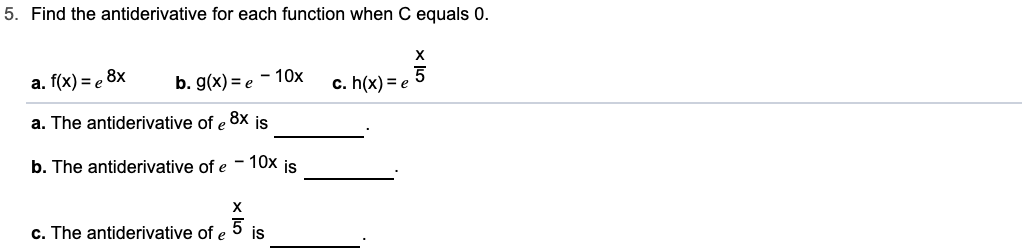# 5. Find the antiderivative for each function when C equals 0.х-10xb. g(x) ea. f(x)e 8xc. h(x) e.8xa. The antiderivative of eisb. The antiderivative of e 10x isх5 isc. The antiderivative of e

Question
14 views

Can i get help step by step with this problem?help_outlineImage Transcriptionclose5. Find the antiderivative for each function when C equals 0. х -10x b. g(x) e a. f(x)e 8x c. h(x) e .8x a. The antiderivative of e is b. The antiderivative of e 10x is х 5 is c. The antiderivative of e fullscreen
check_circle

Step 1

Used the integration formula of e^(mx)

Step 2

b) Use the same formula of e^(mx).

Ans...

### Want to see the full answer?

See Solution

#### Want to see this answer and more?

Solutions are written by subject experts who are available 24/7. Questions are typically answered within 1 hour.*

See Solution
*Response times may vary by subject and question.
Tagged in

### Other